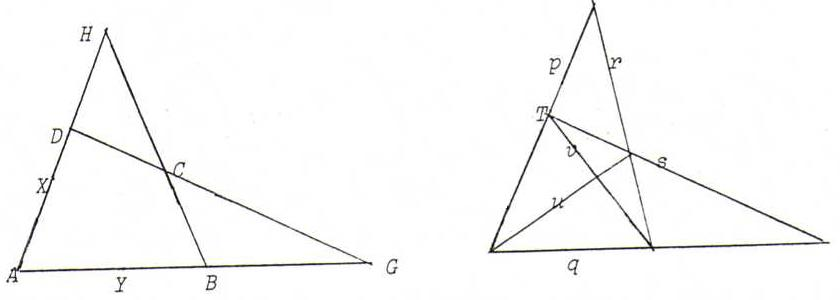### COURSE OF PROJECTIVE GEOMETRY

§ 18:

O71: Repeat O27.

O72: γ = πooγ).

O73: Adapt the proof of the first proposition of §15.

O74H = AD. BC, so v = (p. s)-(q. r); G = AB. CD, so u = (p. q)-(r. s).
X is the fourth harmonic with {A,D} and H, so γ(X) is the fourth harmonic with {p,s} and v.
Likewise, we construct γ(Y).
The intersection point of γ(X) and γ(Y) is γ(l).

Note: X lies on the line connecting G with AC. BD, and on AD, so (alternative construction)
γ(X) goes through the intersection point of u and (p. r)-(q. s), and through p. s.

Do the constructions!